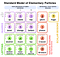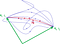# Can you travel faster than the speed of light?

## Light

Light has a peculiar property. It always travels at the same speed, no matter what.

In 1905, Albert Einstein, while discovering the Theory of Relativity, showed that light travelled at only a constant speed. The speed of light is around 300,000 kilometers per second.

Now, Einstein did not prove this or anything, he just postulated it (which means he took it as an reasonably made assumption). Using this postulate, he discovered the theory of relativity: a milestone in physics.

The theory of relativity (which we won’t talk about here much) has been experimentally proven several times. Experiments including high speed muons, light itself, and high energy quarks have shown the theory to be true. So, the postulate was correct indeed.

If you are not comfortable with this, there is another explanation for why light travels at the same speed. Light consists of photons. Photons are subatomic particles.The photon can be seen in the region labelled ‘GAUGE BOSONS’. Source: Wikipedia

Now, photons do not have mass. And according to relativity, any particle or object which has no mass, has to travel at the speed of light. This holds irrespective of whether it is a photon or a gluon (see above).

So, since photons photons have no mass, they travel only at the speed of light. And they cannot change their speed.

## The Relativity Approach:

Now, we answer the question: can we travel faster than light? First, we will look at what relativity has to say.

Relativity is the theory of very fast moving objects. In short, relativity tells us that objects going at near light speeds eventually get thinner and thinner and time passes more slowly for them. This is something we can get to geometrically, but we will not do that here.

Instead, what we are interested in is one small trinket:

Almost everybody may recognize this equation: E = mc². In fact this is not quite true, or rather not quite complete. It actually includes a factor which is usually ignored.

I will not show the equation here explicitly. But, that additional factor basically tells us that as speed increases, our inertia does too.

For those of you who don’t know what inertia is, here is a quick reminder: inertia means the tendency of an object to not change its motion. Objects want to stay as they are, and they resist change. Inertia is a measure of that resistivity. It is directly proportional to the mass.

Now, if you have high inertia, then you have to apply more force, or you have to use more energy to speed it up (or to slow it down).

## Positive mass

We saw that objects which have no mass have to travel at the speed of light. Similarly, then, relativity tells us that objects which do have mass, have to travel at speeds lower than the speed of light.

In an earlier article, we covered how particles get mass: the Higgs mechanism is loosely speaking, a way to slow down particle from the speed of light.

So, now we have established that relativity tells us that massive objects travel at sub-light speed and massless one travel at the speed of light.

So, if you try to speed up a massive particle by applying a force, it’s inertia will increase. If you put some more force, the inertia will further increase.

The point is: as you try to accelerate an object up to the speed of light, by adding more and more force, a) it will require infinite energy, b) it will gain infinite inertia, meaning it will be impossible to move it further.

There are certain laws called conservation laws which says that the amount of energy and mass cannot increase in the universe. So, accordingly getting infinite energy or infinite inertia is just impossible.

So, thus we have solved the problem. Verdict: We cannot travel faster than light, or even reach the speed of light (unless you are massless).

## Appendix: What’s wrong with infinity

Infinity s one of the things that makes physicists go mad. Why? What happen if one single infinity shows up in a solution?

Let’s examine with the help of an example: Feynman Diagrams

Feynman diagrams are used to model interactions between particles. In the above example, we see two electrons which interact with each other using a photons. this makes them repel each other.

Now each part of this diagram is actually a representation of a long, complex integral. When we solve the integral we get a mathematical description of this interaction.

Now, the problem is that this interaction can take place in several ways. If we add together all of theses multiples ways together, then we would get an infinite number of solutions.

So, the math is giving us infinity as a solution for this very interaction. But infinite interactions require infinite energy, and our universe clearly does not have infinite energy.

What happens if our universe has infinite energy? Well, exactly what happens if our universe has infinite anything. Infinite energy means that no meaningful transfer of energy can take place.

Any system in physics, be it a cooling cup of coffee, or a electron orbiting a nucleus, strives towards having as less energy as it can. This is one of the most fundamental principles of physics. If instead, there is an infinite amount of energy, speaking about the lowest within it makes no sense.Everything wants to take the “least energy” to do something. This is an example where a particle takes the path which would require it using the least energy, shown in red, while it could have taken any of the other paths shown. Source: Wikipedia

Whenever infinity shows up in any calculation, we know there has to be something wrong in it. We can’t find infinity in real nature (most of the time), so we need to modify our theory.

The infinite energy result we got: keep it in your mind we will need it later. As for the case shown above (the Feynman diagrams one), we solve the problem using renormalization, which we may see at later times.

## But wait.. what about Tachyons?

Tachyons are a theoretical type of particles which can supposedly travel faster than the speed of light.

How? It requires a slight modification. And do they really travel faster than the speed of light? Yes, but not really.

We will discuss tachyons, harmonic oscillators, ground states, the Higgs vacuum and one more way to destroy the universe in the sequel. Coming soon, on Einstein’s Cup Of Tea.

Written by

Written by

## Pravit Shetty

#### An ever enthusiastic reader, physics nerd, math geek, and amateur writer.